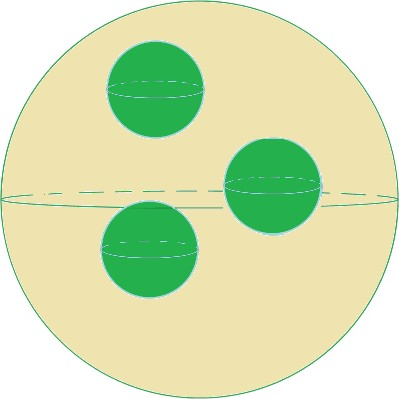Surface Area and Volume of Spheres

Surface Area and Volume of Spheres

A sphere is a 3-D figure in which all of the points in a plane are the same distance from a given point, the center of the sphere.

The diameter of the sphere is the straight line that connects two points on the sphere’s surface while passing through the center.

The radius of the sphere is a straight line from the center of the sphere to any point on the surface of the sphere.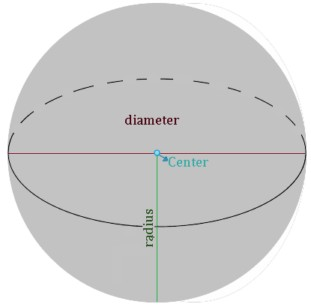Surface area of a sphere

The surface area of a sphere with radius r is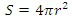or using diameter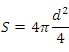S =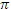d 2

Example

A spherical ball is inflated to 4 times its original radius. If the original radius was 4 cm, would the surface area quadruple as well?

Solution

For the original sphere with a radius r, we can calculate the surface area: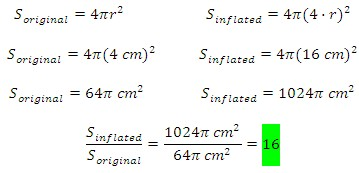The surface area of the inflated sphere is 16 times the surface area of the original, so it does NOT quadruple.

Volume of a sphere

Taking a look at the image below, we can picture the interior of a sphere. Its radius r is approximated by n pyramids, each of them with a height equal to the radius of the sphere and a defined base.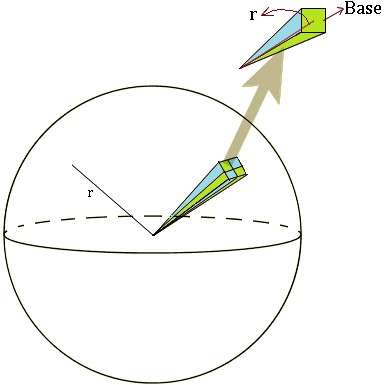We can use this formula to find the volume of the pyramid: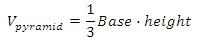Since we have n amount of pyramids that represent the volume inside the sphere, the surface area for the sphere is defined as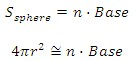We can approximate the volume of the sphere by combining the equations adding all of the n pyramids inside the sphere.  This gives us: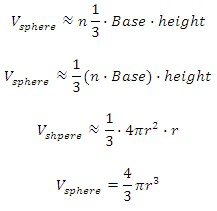Example

You are given a clay prism (see below) and are told to create a ball using all the clay. What will be the radius of the ball you create?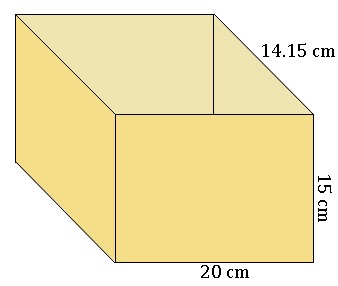Solution

To find the volume of the prism we have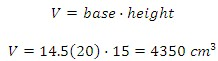Since they clay ball and prism will have the same volume, we can use the volume formula for the sphere to find the radius.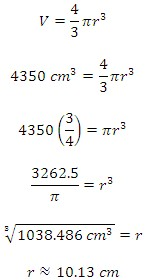The radius of the ball is then approximately 10.1 centimeters.

Practice.  Round answers to the nearest hundredth.

1. Find the surface area: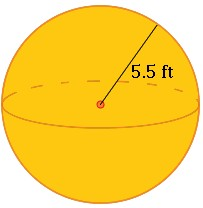2.) Find the volume of the sphere below: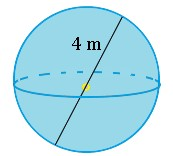3.) If three small spheres each have a radius of 250 cm, and the large sphere has a surface area of 2,500 m2, how much of the volume of the big sphere is not occupied by the small spheres?